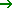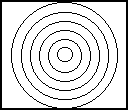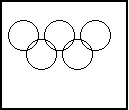Tutorial 16: Circles
Drawing Circles on the Graph Screen...
 HOME >> Tutorials >> Tutorial 16: Circles

Introduction:
Unlike lines, there is no easy way to make a circle without using the command that draws a circle. In this tutorial there will not be many sets of code because there aren't many ways to use a circle without making a picture and using other commands like lines, pixels, and points. This tutorial only has one command:

New Commands:

 Circle( Draws a circle with the center at (x,y) and a radius

The Codes:
There are only going to be two codes this time. The first will show you how to draw circles inside of other circles and the second will draw the Olympics 5-ring symbol.

 : For AShell, SOS, and TI-Explorer AxesOff Turns the graph axes off FnOff Deselects all the Y= functions 0Xmin Stores Xmin as 0 94Xmax Stores Xmax as 94 0Ymax Stores Ymax as 0 -62Ymin Stores Ymin as -62 ClrDraw Clears the graph screen of all drawings Circle(47,-31,30 Draws a circle centered at (47,-31) and radius of 30 Circle(47,-31,5 Draws a circle centered at (47,-31) and radius of 5 Circle(47,-31,25 Draws a circle centered at (47,-31) and radius of 25 Circle(47,-31,10 Draws a circle centered at (47,-31) and radius of 10 Circle(47,-31,20 Draws a circle centered at (47,-31) and radius of 20 Circle(47,-31,15 Draws a circle centered at (47,-31) and radius of 15 Pause Suspends program and waits for user to pressClrDraw Clears the graph screen of all drawings For(X,5,30,5 X stored from 5-30 with increments of 5 Circle(47,-31,X Draws a circle at (47,-31) and radius of X value End End the For looppressAs you may have already noticed, this program takes a long time to finish because it draws the circles so slowly, but there's nothing we can do about that. Notice how the same thing is displayed before and after the Pause. You can do it both ways, but in this case, I would prefer to do it the second way because it saves space. Neither way makes the program any faster, but the second way saves the bytes. Next, we'll make the Olympics logo.

 : For AShell, SOS, and TI-Explorer AxesOff Turns the graph axes off FnOff Deselects all the Y= functions 0Xmin Stores Xmin as 0 94Xmax Stores Xmax as 94 0Ymax Stores Ymax as 0 -62Ymin Stores Ymin as -62 ClrDraw Clears the graph screen of all drawings Circle(15,-15,12 Draws a circle centered at (15,-15) and radius of 12 Circle(30,-30,12 Draws a circle centered at (30,-30) and radius of 12 Circle(45,-15,12 Draws a circle centered at (45,-15) and radius of 12 Circle(60,-30,12 Draws a circle centered at (60,-30) and radius of 12 Circle(75,-15,12 Draws a circle centered at (75,-15) and radius of 12Pretty cool, huh? It actually came out pretty nice. It took a couple of trials and error to get it exactly right (at first I had a radius of 20 and then a radius of 15), but I finally got it.

Conclusion:
I think that should be enough for you to grasp how to use Circle(. I can't really think of any other ways to show you how to use it. I was going to show you how to make a coke can, but I'm leaving that for the tutorial on draw functions. The next tutorial is on shading, which is the final step in making good pictures.

If you do not understand a particular part in this lesson, have suggestions, or find any problems please contact me.